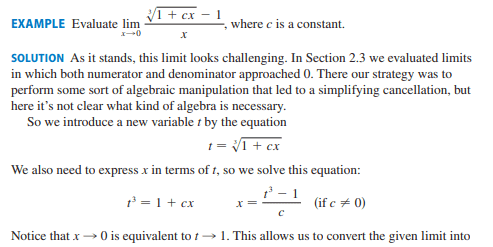# Limit of a rational function with a constant c

Callumnc1
Homework Statement:
Relevant Equations:
For this problem,Did they get ## x## approaches one is equivalent to ##t## approaches zero because ##t ∝ (x)^{1/3} + 1##?

Many thanks!

Last edited:

Gold Member
" Notice that x ##\rightarrow##0 is equivalent to t ##\rightarrow## 1 ", it says. The given formula becomes
$$\lim_{t \rightarrow 1} \frac{c(t-1)}{t^3-1}$$

•Callumnc1
Callumnc1
" Notice that x ##\rightarrow##0 is equivalent to t ##\rightarrow## 1 ", it says. The given formula becomes
$$\lim_{t \rightarrow 1} \frac{c(t-1)}{t^3-1}$$

Sorry, I have updated the question after I realized my mistake. Is my reasoning correct though?

Many thanks!

Gold Member
$$\lim_{x\rightarrow 0}t=\lim_{x\rightarrow 0} \sqrt{1+cx}=1$$
$$\lim_{t\rightarrow 1}x=\lim_{t\rightarrow 1}\frac{t^3-1}{c}=0$$

•Callumnc1
Staff Emeritus
Homework Helper
Gold Member

For this problem,
View attachment 322282
Did they get ## x## approaches one is equivalent to ##t## approaches zero because ##t ∝ (x)^{1/3} + 1##?

Many thanks!
I would say it's more like:

As ##x\to 0##, it's clear that ##\displaystyle \root 3 \of{1+cx \,} \to 1##, so ##t\to 1## .

Last edited:
•Callumnc1
Callumnc1
$$\lim_{x\rightarrow 0}t=\lim_{x\rightarrow 0} \sqrt{1+cx}=1$$
$$\lim_{t\rightarrow 1}x=\lim_{t\rightarrow 1}\frac{t^3-1}{c}=0$$

Callumnc1
I would say it's more like:

As ##x\to 0##, it's clear that ##\root 3 \of{1+cx \,} \to 1##, so ##t\to 1## .
Thank you @SammyS , I see now!

Staff Emeritus
Homework Helper
Is my reasoning correct though?
It looks like you're thinking ##\sqrt{1+cx} = \sqrt{1} + \sqrt{cx}##. That's clearly wrong. You can't distribute the root across the addition.

Also, as far as notation goes, ##\propto## means "proportional to", so saying that ##t \propto 1 + x^{1/3}## means that ##t = k(1+x^{1/3})## for some constant ##k##, which you probably didn't mean.

•Callumnc1 and PeroK
Callumnc1
It looks like you're thinking ##\sqrt{1+cx} = \sqrt{1} + \sqrt{cx}##. That's clearly wrong. You can't distribute the root across the addition.

Also, as far as notation goes, ##\propto## means "proportional to", so saying that ##t \propto 1 + x^{1/3}## means that ##t = k(1+x^{1/3})## for some constant ##k##, which you probably didn't mean.